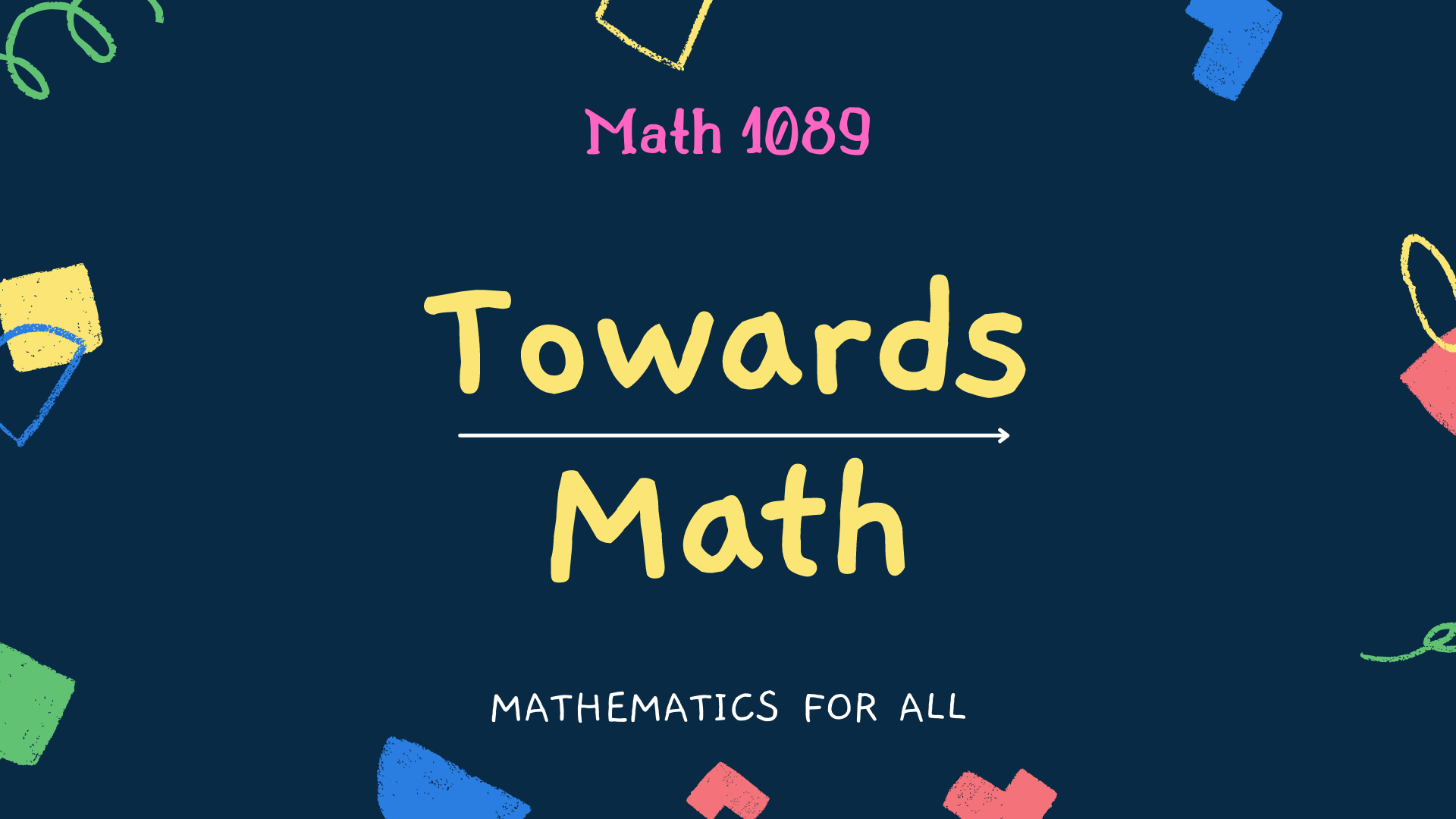# Amazing Number Circle

Below we will consider a few numbers arranged in a circle. The numbers are first 32 natural numbers, i.e., 1, 2, 3, . . . , 31, 32. The numbers on the circle are so arranged that the sum of any two consecutive numbers is a perfect square. While adding two consecutive numbers, either we get 9 = 32 or 16 = 42 or 25 = 52 or 36 = 62 or 49 = 72.

21 + 4 = 25 = 52

4 + 32 = 36 = 62

32 + 17 = 49 = 72

17 + 19 = 36 = 62

19 + 30 = 49 = 72

30 + 6 = 36 = 62

6 + 3 = 9 = 32

3 + 13 = 16 = 42

13 + 12 = 25 = 52

12 + 24 = 36 = 62

24 + 25 = 49 = 72

25 + 11 = 36 = 62

11 + 5 = 16 = 42

5 + 31 = 36 = 62

31 + 18 = 49 = 72

18 + 7 = 25 = 52

7 + 29 = 36 = 62

29 + 20 = 49 = 72

20 + 16 = 36 = 62

16 + 9 = 25 = 52

9 + 27 = 36 = 62

27 + 22 = 49 = 72

22 + 14 = 36 = 62

14 + 2 = 16 = 42

2 + 23 = 25 = 52

23 + 26 = 49 = 72

26 + 10 = 36 = 62

10 + 15 = 25 = 52

15 + 1 = 16 = 42

1 + 8 = 9 = 32

8 + 28 = 36 = 62

28 + 21 = 49 = 72

Numbers are beautiful!

1.Sandeep kumar says:

Very interesting sir.

1.Math1089 says:

Thank you Sir

2.StayCalm says:

37 itself is interesting, and so are 37037, 37037037, etc. for similar reasons.

1.Math1089 says:

These are in pipeline. Thank you for your suggestion.

2.souravkumarbejgmailcom says:

Wow…like this we can create more square numbers!!

1.Math1089 says:

Thank you Sourav.

3.Alex Marshall says:

Wow! Next time around, it’s Math for me!

1.Math1089 says:

Good to hear this. Thank you so much.

4.Carey Wrye says:

1.Math1089 says: